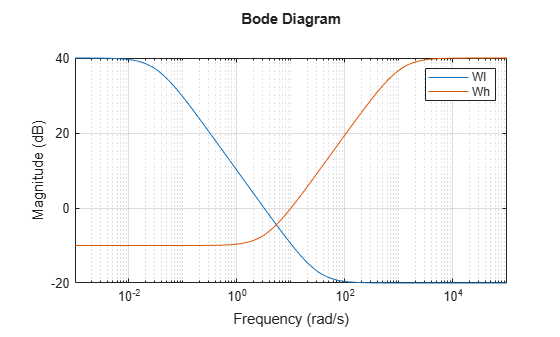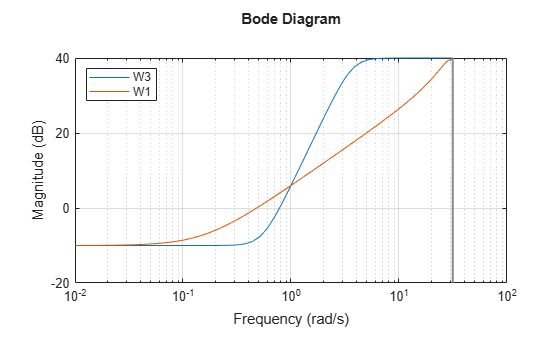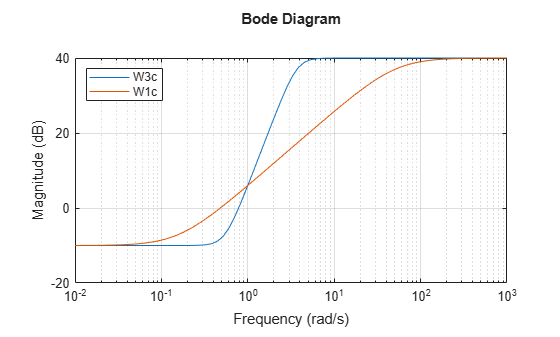# makeweight

Weighting function with monotonic gain profile

## Syntax

`` W = makeweight(dcgain,[freq,mag],hfgain)``
``W = makeweight(dcgain,[freq,mag],hfgain,Ts)``
``W = makeweight(dcgain,[freq,mag],hfgain,Ts,N)``
``W = makeweight(dcgain,wc,hfgain,___)``

## Description

`makeweight` is a convenient way to specify loop shapes, target gain profiles, or weighting functions for applications such as controller synthesis and control system tuning.

example

```` W = makeweight(dcgain,[freq,mag],hfgain)` creates a first-order, continuous-time weight W(s) satisfying these constraints:$\begin{array}{c}W\left(0\right)=\text{dcgain}\\ W\left(\text{Inf}\right)=\text{hfgain}\\ |W\left(j\cdot \text{freq}\right)|=\text{mag}\text{.}\end{array}$In other words, the gain of `W` passes through `mag` at the finite frequency `freq`. ```

example

````W = makeweight(dcgain,[freq,mag],hfgain,Ts)` creates a first-order, discrete-time weight W(z) satisfying these constraints:$\begin{array}{c}W\left(1\right)=\text{dcgain}\\ W\left(-1\right)=\text{hfgain}\\ |W\left({e}^{j\cdot \text{freq}\cdot \text{Ts}}\right)|=\text{mag}\text{.}\end{array}$In other words, the gain of `W` passes through `mag` at the frequency `freq`. The frequency `freq` must satisfy 0 < `freq` < π/`Ts`. ```

example

````W = makeweight(dcgain,[freq,mag],hfgain,Ts,N)` uses an `N`th-order transfer function with poles and zeros in a Butterworth pattern to meet the constraints. The higher the order `N`, the steeper the transition from low to high gain. To create a continuous-time higher order weighting function, use `Ts` = 0.```

example

````W = makeweight(dcgain,wc,hfgain,___)` specifies the gain crossover frequency `wc`. This syntax is equivalent to setting `[freq,mag]` to `[wc,1]`. You can use this syntax with any of the previous input-argument combinations to create a continuous-time, discrete-time, or Butterworth weighting function.```

## Examples

collapse all

Create continuous-time weighting functions by specifying the low-frequency gain, high-frequency gain, and magnitude of the gain at some intermediate frequency.

For instance, create a weighting function with a gain of 40 dB at low frequency, rolling off to –20 dB at high frequency. Specify further that the gain is about 10 dB at 1 rad/s by putting these values in a vector `[freq,mag]`. Specify all the gains in absolute units.

`Wl = makeweight(100,[1,3.16],0.1);`

Create a weighting function with a gain of –10 dB at low frequency, rising to 40 dB at high frequency. Specify a 0 dB crossover frequency of 10 rad/s. To specify a 0 dB crossover frequency, you can use the crossover frequency as the second input argument instead of the vector `[freq,mag]`.

`Wh = makeweight(0.316,10,100);`

Plot the magnitudes of the weighting functions to confirm that they meet the response specifications.

```bodemag(Wl,Wh) legend grid on```Create a gain profile that rolls off at high frequency without flattening. Specify a gain of 40 dB at low frequency and a crossover frequency of 10 rad/s.

`W = makeweight(100,[10 1],0);`

Specifying a high-frequency gain of 0 ensures that the frequency response rolls off at high frequencies without leveling off. Plot the gain profile to confirm this shape.

```bodemag(W) grid on```Create discrete-time weighting functions by specifying the low-frequency gain, high-frequency gain, magnitude of the gain at some intermediate frequency, and sample time.

Create a weighting function with a sample time of 0.1 s. Specify a gain of 40 dB at low frequency, rolling off to –20 dB at high frequency. Specify further that the gain is about 10 dB at 0.01 rad/s. Provide all gains in absolute units.

`Wl = makeweight(100,[0.01,3.16],0.1,0.1);`

Create a weighting function with a gain of –10 dB at low frequency, rising to 40 dB at high frequency. Specify a 0 dB crossover frequency of 2 rad/s and a sample time of 0.1 s. To specify a 0 dB crossover frequency, you can use the crossover frequency as the second input argument instead of the vector `[freq,mag]`.

`Wh = makeweight(0.316,2,100,0.1);`

Plot the magnitudes of the weighting functions to confirm that they meet the response specifications.

```bodemag(Wl,Wh) grid on```The high-frequency leveling of `Wh` is distorted due to the proximity of its crossover frequency to the Nyquist frequency.

By default, `makeweight` creates first-order weighting functions. If you want a sharper transition between the low-frequency and high-frequency gains, you can specify the order with the last input argument. For instance, suppose you want to create a weighting function with a sample time of 0.1 s. The function has a gain of –10 dB at low frequency, rising to 40 dB at high frequency. Additionally, the gain passes through 6 dB at 1 rad/s. For comparison, create both a third-order and a first-order function with these specifications.

```W3 = makeweight(0.316,[1 2],100,0.1,3); W1 = makeweight(0.316,[1 2],100,0.1); bodemag(W3,W1) legend('location','northwest') grid on```For the first-order function, the high-frequency leveling is distorted due to the proximity of its crossover frequency to the Nyquist frequency. Using a sharper, higher-order transition ensures that the function has leveled out before reaching the Nyquist frequency.

To create continuous-time weighting functions of higher order, set `Ts = 0`. For instance, create continuous-time weighting functions with the same gain specifications as `W1` and `W3`.

```W3c = makeweight(0.316,[1 2],100,0,3); W1c = makeweight(0.316,[1 2],100); bodemag(W3c,W1c) legend('location','northwest') grid on```## Input Arguments

collapse all

Low-frequency gain of the weighting function, specified as a real scalar value. Express the gain in absolute units. For example, to specify a low-frequency gain of 20 dB, set `dcgain` = 10.

The low-frequency gain, high-frequency gain, and magnitude must satisfy:

• |`dcgain`| > `mag` > |`hfgain`| for a low-pass weight

• |`dcgain`| < `mag` < |`hfgain`| for a high-pass weight

Target magnitude and corresponding frequency, specified as a two-element vector. You specify where the gain of `W` transitions between the low-frequency and high-frequency values by specifying a target magnitude at a particular frequency. For instance, if you set `[freq,mag] = [10,0.1]`, then the magnitude of `W` passes through 0.1 (–10 dB) at a frequency of 10 rad/s. Similarly, setting `[freq,mag] = [5,1]` specifies a 0 dB (unit gain) crossover frequency of 5 rad/s.

The low-frequency gain, high-frequency gain, and magnitude must satisfy:

High-frequency gain of the weighting function, specified as a real scalar value. Express the gain in absolute units. For example, to specify a high-frequency gain of –20 dB, set `dcgain` = 0.1.

The low-frequency gain, high-frequency gain, and magnitude must satisfy:

• |`dcgain`| > `mag` > |`hfgain`| for a low-pass weight

• |`dcgain`| < `mag` < |`hfgain`| for a high-pass weight

Sample time of discrete-time weighting function, specified as a nonnegative scalar value or –1. A positive value sets the sample time in seconds. The special value –1 creates a discrete-time state-space model with an unspecified sample time.

Setting `Ts` = 0 creates a continuous-time weighting function. This value is useful when you want to create higher order continuous-time transfer functions using the `N` input argument. For an example, see Higher Order Weighting Functions.

Order of weighting function, specified as a positive integer. `makeweight` uses an `N`th-order transfer function with poles and zeros in a Butterworth pattern to meet the specified gain constraints. The higher the order `N`, the steeper the transition from low to high gain.

Crossover frequency of the weighting function in radians/second, specified as a positive scalar value. Using the input argument `wc` is equivalent to using `[freq,mag]` = `[wc,1]`.

For discrete-time weighting functions, the crossover frequency must satisfy `wc*Ts` < π.

## Output Arguments

collapse all

Weighting function, returned as a state-space (`ss`) model. For continuous-time weighting functions, the response of `W` satisfies the following:

`$\begin{array}{c}W\left(0\right)=\text{dcgain}\\ W\left(\text{Inf}\right)=\text{hfgain}\\ |W\left(j\cdot \text{freq}\right)|=\text{mag}\text{.}\end{array}$`

For discrete-time weighting functions, the response of `W` satisfies the following:

`$\begin{array}{c}W\left(1\right)=\text{dcgain}\\ W\left(-1\right)=\text{hfgain}\\ |W\left({e}^{j\cdot \text{freq}\cdot \text{Ts}}\right)|=\text{mag}\text{.}\end{array}$`

## Version History

Introduced before R2006a